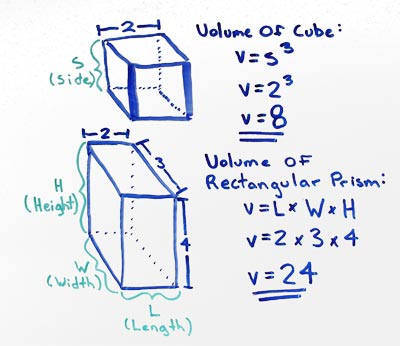# Volume of Cube

## Simple Cube Volume

A cube with a side length (S) of 2 has a volume (V) of 8.

## Rectangular Prism Volume

A rectangular prism with a length (L) of 2 a width (W) of 3 and a height (W) of 4 has a volume (V) of 24.A cube has three equal sides (S), this means the volume can be determined by multiplying Side x Side x Side, this is the same as Side3.

If one of the sides is not the same then this is a rectangular prism. The volume of a rectangular prism can be determined by multiplying Length (L) x Width (W) x Height (H).

### Formulas

What is the formula for the volume of a Cube?

V = S3

What is the formula for the volume of a Rectangular Prism?

V = L x W x H

### Other Formulae

for cubes with side length S

Cube Surface Area

6S2
Cube Face Diagonal
(√2)S
Cube Space Diagonal
(√3)S
S/2
All formulae...